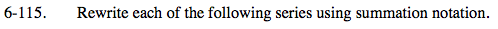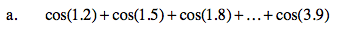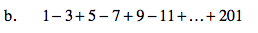### Home > PC > Chapter 6 > Lesson 6.3.1 > Problem6-115

6-115.$\sum_{k=0}^{\text{terms}-1}\text{cos(pattern)}$What expression would change the sign back and forth (+ to −)?

(−1)n
n is an integer.

What expression gives odd numbers?

2n + 1